# COLLIGATIVE PROPERTIES

Colligative properties of solutions are properties that depend upon the concentration of solute molecules or ions, but not upon the identity of the solute. Colligative properties include vapor pressure lowering, boiling point elevation, freezing point depression, and osmotic pressure.

Lowering the Vapor Pressure:

Let’s first remind ourselves about what vapor pressure is and what affects it chemically and physically. In a closed container, a liquid will evaporate until an equal amount of molecules are returning to the liquid state as there are escaping into the gas phase. The pressure of the vapor phase above the liquid at this point is called the equilibrium vapor pressure.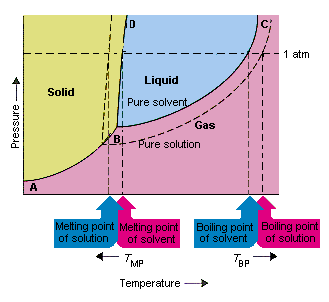This vapor pressure is dependent on a number of factors, including the temperature of the system (kinetic energy is required to help the molecules escape into the gas phase), the pressure of the system (high pressure can keep the gas contained in the liquid e.g. a bottle of coke), and also the intermolecular forces of the liquid itself (stronger bonds will require more kinetic energy to break slowing the evaporation process, lowering the overall vapor pressure of the system).

What happens to the system if we add a solute to the solvent in question? Well, in the simplest terms, since some of the solute molecules will take up spaces at the surface of the liquid, this will limit the number of solvent molecules at the surface.

Since only solvent molecules located at the surface can escape (evaporate), the sheer presence of the solute lowers the number of solvent molecules coming and going and therefore lowers the equilibrium vapor pressure. In order to figure out how much the solute has affected the vapor pressure, we need to approximate how many fewer molecules are able to reach the surface of the liquid, correct? A good approximation of how many molecules there are of solvent versus solute is the mole fraction, Xsolvent.

If we simply multiply the new mole fraction of solvent by the standard vapor pressure (Posolvent) of the pure solvent, this will give us a good approximation of the new vapor pressure of the solvent. The equation would look like Psolvent = Xsolvent Posolvent.

This equation is called Raoult’s Law. Note that the pressure you obtain from this equation is the new equilibrium vapor pressure of the system with the solute included.

If you want to know how much change has taken place, you will need to look at the difference between the new Psolventand the original Posolvent of the pure solvent (in other words subtract one from the other) DP = Psolvent – Posolvent = change in the vapor pressure of the system. For almost all non-volatile solvents, this change will be a negative value.

### Limitations on Raoult’s Law:

Raoult’s law only works for low concentration solutions. Why? Well, in order for our approximation to work, the interactions between the solute and solvent molecules must be nearly identical. If the interactions are stronger, then the heat of vaporization of the solvent will change and thus our whole approximation falls apart. Since we know that intermolecular forces vary greatly between molecules, the affect of those forces must be kept at a minimum by keeping the solute concentration real low. You can think of it as having one stinky guy at a party in a room of 1000 people, there is a very good chance that you won’t have to smell him, but if there were 100 stinky guys in the room, it would ruin the whole party, right?

Another consideration is the type of solute. If the solute is an ionic species (a salt) then it is most likely going to split up into its component ions when it hits the solvent (normally water). If this is the case, we need to consider each particle that forms. For instance, if you added 0.25 moles of NaCl to a solution, you would actually be adding 0.25 moles of Na+ and 0.25 moles of Cl for an overall colligative affect of 0.50 moles of ions. This value of 2 x 0.25 is called the van’t Hoff factor (i) and must be used whenever you calculate colligative properties of solutions containing ions.

### Boiling Point Elevation:

Now that we have seen how addition of solutes to a solvent can lower its vapor pressure, let’s see if we can figure out how this relates to the boiling point of the same solvent.

The normal boiling point of a liquid is defined as the temperature at which the vapor pressure of the liquid is equal to standard pressure (1 atm). If we change the external pressure from 1 atm lower or higher, the boiling point changes as well. The boiling point is then simply the temperature at which the vapor pressure of the solvent equals the pressure of the surroundings.

If you think about it, up until the vapor pressure reaches this point, the gas molecules cannot escape, right? The pressure on the surface is holding them in. Once you reach the boiling point temperature, the vapor pressure is then equal to the external pressure and the molecules can escape. Note that you really have to exceed the boiling point temperature to fully evaporate the liquid. I.e. steam is hotter than 100oC.

Now back to colligative properties…how does adding solutes to a solvent affect the boiling point? Well, we now understand that solute molecules taking up positions at the surface of the liquid will depress the vapor pressure. We also know that we have to raise the vapor pressure of the liquid in order to reach the boiling point so this must mean we will have to input more energy to reach the same vapor pressure. Input of more energy equates to the input of heat in this type of system, so the boiling point temperature must be higher to obtain the same vapor pressure. Adding a non-volatile solute to a solution therefore raises the boiling point of that solution.

When a solute is dissolved in a solvent, the boiling point of the solution is raised according to the equation:

DT = iKbm

DT= change in temperature
= the van’t Hoff factor, which is the number of particles into which the solute dissociates
m = the molality, which is the moles of solute per kilograms of solvent
Kb = the molal boiling point constant (for water, Kb = 0.5121oC/m)

### Freezing Point Depression:

Let’s start this discussion the same way we started the others, by defining the normal freezing point. The normal freezing point of a liquid is is the temperature at which a liquid becomes a solid at 1 atm. A more specific definition of freezing point is the temperature at which solid and liquid phases coexist in equilibrium. Let’s see if we can figure out why the freezing point is lowered when we add solutes to a solution. We already know that in order to freeze a liquid, we have to lower the temperature. As the temperature lowers, the solution becomes more ordered as it moves toward the solid phase. This is an effect that works against the second law of thermodynamicsIn short, entropy (disorder) likes to increase not decrease in the natural scheme of things. So if we have to lower the temperature to a certain point to freeze a pure solvent, when we add a solute we add to the entropy of the system, right? The mixture is more disordered than the pure. This additional amount of entropy must now be overcome to allow the liquid to change phases into a solid (become ordered). This means that the temperature will have to be even lower than before. Thus addition of any type of solute to a solvent will lower its freezing point.

As with boiling point elevation, the equation to figure out how great the change in temperature will be is given by:

DT = iKfm

DT= change in temperature
= the van’t Hoff factor, which is the number of particles into which the solute dissociates
m = the molality, which is the moles of solute per kilograms of solvent
Kf = the molal freezing point constant (for water, Kf = -1.86 oC/m)

Using colligative properties to determine molecular weight of a substance:

Colligative properties such as freezing point depression or boiling point elevation can be used to calculate the molecular weight of a soluble solid.  To complete this calculation, the mass of solute and solvent must be known as well as the freezing points/boiling points of the pure solvent and the solution.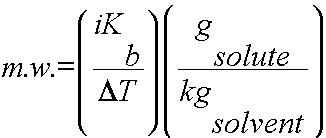or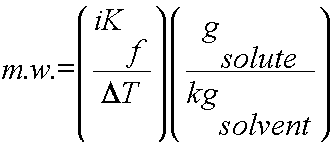Here are the steps to take:

1) Determine DT

2) Use DT, i and Kb or Kf to determine the molality of the solute (m = gsolute/kgsolvent).

3) Plug in all values and calculate for the m.w.

4) Use your common sense to assess your calculated value as reasonable or not! In other words, check for boo-boos.

Note: You can also use vapor pressure change or osmosis in the same manner to calculate molecular weight.

# Mole Fraction and Molality

Several units commonly used to express the concentrations of solution components were introduced in an earlier chapter of this text, each providing certain benefits for use in different applications. For example, molarity (M) is a convenient unit for use in stoichiometric calculations, since it is defined in terms of the molar amounts of solute species:$M = \frac{\text{mol\;solute}}{\text{L\;solution}}$

Because solution volumes vary with temperature, molar concentrations will likewise vary. When expressed as molarity, the concentration of a solution with identical numbers of solute and solvent species will be different at different temperatures, due to the contraction/expansion of the solution. More appropriate for calculations involving many colligative properties are mole-based concentration units whose values are not dependent on temperature. Two such units are mole fraction (introduced in the previous chapter on gases) and molality.

The mole fraction, X, of a component is the ratio of its molar amount to the total number of moles of all solution components:$X_{\text{A}} = \frac{\text{mol\;A}}{\text{total\;mol\;of\;all\;components}}$

Molality is a concentration unit defined as the ratio of the numbers of moles of solute to the mass of the solvent in kilograms:$m = \frac{\text{mol\;solute}}{\text{kg\;solvent}}$

Since these units are computed using only masses and molar amounts, they do not vary with temperature and, thus, are better suited for applications requiring temperature-independent concentrations, including several colligative properties, as will be described in this chapter module.

# Vapor Pressure Lowering

As described in the chapter on liquids and solids, the equilibrium vapor pressure of a liquid is the pressure exerted by its gaseous phase when vaporization and condensation are occurring at equal rates:$\text{liquid}\;{\leftrightharpoons}\;\text{gas}$

Dissolving a nonvolatile substance in a volatile liquid results in a lowering of the liquid’s vapor pressure. This phenomenon can be rationalized by considering the effect of added solute molecules on the liquid’s vaporization and condensation processes. To vaporize, solvent molecules must be present at the surface of the solution. The presence of solute decreases the surface area available to solvent molecules and thereby reduces the rate of solvent vaporization. Since the rate of condensation is unaffected by the presence of solute, the net result is that the vaporization-condensation equilibrium is achieved with fewer solvent molecules in the vapor phase (i.e., at a lower vapor pressure) (Figure 1). While this kinetic interpretation is useful, it does not account for several important aspects of the colligative nature of vapor pressure lowering. A more rigorous explanation involves the property of entropy, a topic of discussion in a later text chapter on thermodynamics. For purposes of understanding the lowering of a liquid’s vapor pressure, it is adequate to note that the greater entropy of a solution in comparison to its separate solvent and solute serves to effectively stabilize the solvent molecules and hinder their vaporization. A lower vapor pressure results, and a correspondingly higher boiling point as described in the next section of this module.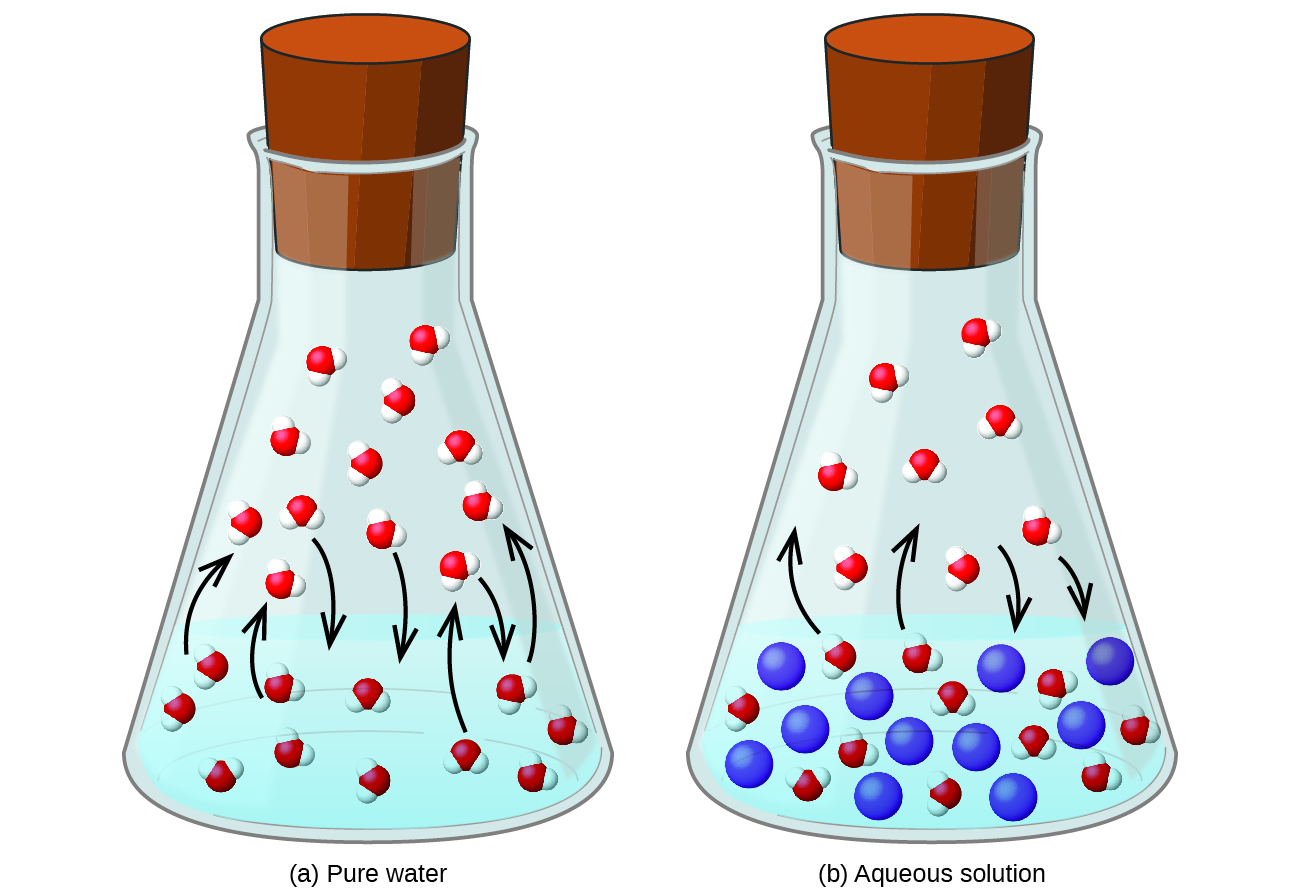Figure 1. The presence of nonvolatile solutes lowers the vapor pressure of a solution by impeding the evaporation of solvent molecules.

The relationship between the vapor pressures of solution components and the concentrations of those components is described by Raoult’s lawThe partial pressure exerted by any component of an ideal solution is equal to the vapor pressure of the pure component multiplied by its mole fraction in the solution.$P_{\text{A}} = X_{\text{A}}P_{\text{A}}^{\circ}$

where PA is the partial pressure exerted by component A in the solution,$P_{\text{A}}^{\circ}$ is the vapor pressure of pure A, and XA is the mole fraction of A in the solution. (Mole fraction is a concentration unit introduced in the chapter on gases.)

Recalling that the total pressure of a gaseous mixture is equal to the sum of partial pressures for all its components (Dalton’s law of partial pressures), the total vapor pressure exerted by a solution containing i components is$P_{\text{solution}} = \sum\limits_{i}\;P_i = \sum\limits_{i}\;X_iP_i^{\circ}$

A nonvolatile substance is one whose vapor pressure is negligible (P° ≈ 0), and so the vapor pressure above a solution containing only nonvolatile solutes is due only to the solvent:$P_{\text{solution}} = X_{\text{solvent}}P_{\text{solvent}}^{\circ}$

# Elevation of the Boiling Point of a Solvent

As described in the chapter on liquids and solids, the boiling point of a liquid is the temperature at which its vapor pressure is equal to ambient atmospheric pressure. Since the vapor pressure of a solution is lowered due to the presence of nonvolatile solutes, it stands to reason that the solution’s boiling point will subsequently be increased. Compared to pure solvent, a solution, therefore, will require a higher temperature to achieve any given vapor pressure, including one equivalent to that of the surrounding atmosphere. The increase in boiling point observed when nonvolatile solute is dissolved in a solvent, ΔTb, is called boiling point elevation and is directly proportional to the molal concentration of solute species:${\Delta}T_{\text{b}} = K_{\text{b}}m$

where Kb is the boiling point elevation constant, or the ebullioscopic constant and m is the molal concentration (molality) of all solute species.

Boiling point elevation constants are characteristic properties that depend on the identity of the solvent. Values of Kb for several solvents are listed in Table 2.

Solvent Boiling Point (°C at 1 atm) Kb(Cm−1) Freezing Point (°C at 1 atm) Kf(Cm−1)
water 100.0 0.512 0.0 1.86
hydrogen acetate 118.1 3.07 16.6 3.9
benzene 80.1 2.53 5.5 5.12
chloroform 61.26 3.63 −63.5 4.68
nitrobenzene 210.9 5.24 5.67 8.1
Table 2. Boiling Point Elevation and Freezing Point Depression Constants for Several Solvents

The extent to which the vapor pressure of a solvent is lowered and the boiling point is elevated depends on the total number of solute particles present in a given amount of solvent, not on the mass or size or chemical identities of the particles. A 1 m aqueous solution of sucrose (342 g/mol) and a 1 m aqueous solution of ethylene glycol (62 g/mol) will exhibit the same boiling point because each solution has one mole of solute particles (molecules) per kilogram of solvent.

# Distillation of Solutions

Distillation is a technique for separating the components of mixtures that is widely applied in both in the laboratory and in industrial settings. It is used to refine petroleum, to isolate fermentation products, and to purify water. This separation technique involves the controlled heating of a sample mixture to selectively vaporize, condense, and collect one or more components of interest. A typical apparatus for laboratory-scale distillations is shown in Figure 2.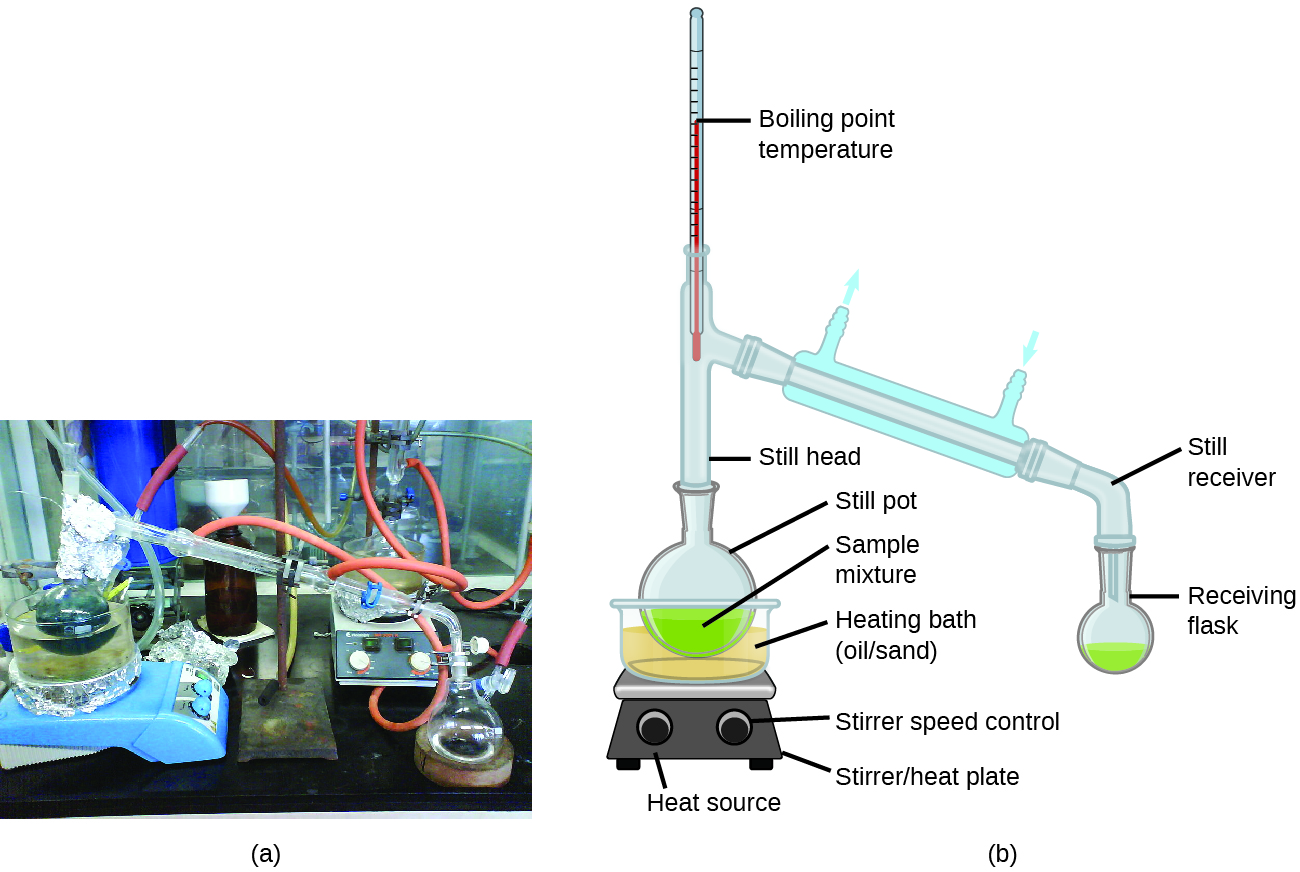Figure 2. A typical laboratory distillation unit is shown in (a) a photograph and (b) a schematic diagram of the components. (credit a: modification of work by “Rifleman82”/Wikimedia commons; credit b: modification of work by “Slashme”/Wikimedia Commons)

Oil refineries use large-scale fractional distillation to separate the components of crude oil. The crude oil is heated to high temperatures at the base of a tall fractionating column, vaporizing many of the components that rise within the column. As vaporized components reach adequately cool zones during their ascent, they condense and are collected. The collected liquids are simpler mixtures of hydrocarbons and other petroleum compounds that are of appropriate composition for various applications (e.g., diesel fuel, kerosene, gasoline), as depicted in Figure 3.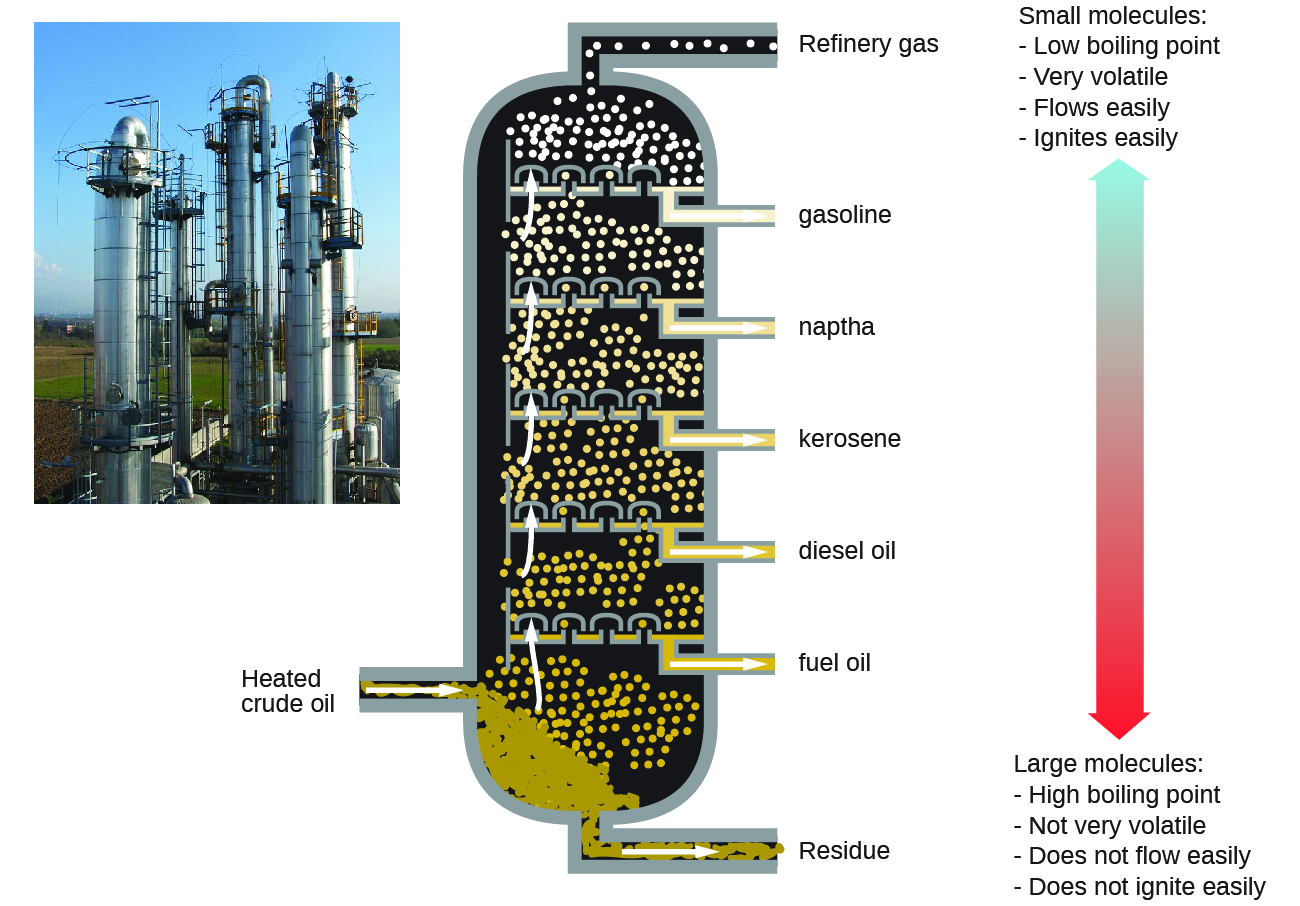Figure 3. Crude oil is a complex mixture that is separated by large-scale fractional distillation to isolate various simpler mixtures.

# Depression of the Freezing Point of a Solvent

Solutions freeze at lower temperatures than pure liquids. This phenomenon is exploited in “de-icing” schemes that use salt (Figure 4), calcium chloride, or urea to melt ice on roads and sidewalks, and in the use of ethylene glycol as an “antifreeze” in automobile radiators. Seawater freezes at a lower temperature than fresh water, and so the Arctic and Antarctic oceans remain unfrozen even at temperatures below 0 °C (as do the body fluids of fish and other cold-blooded sea animals that live in these oceans).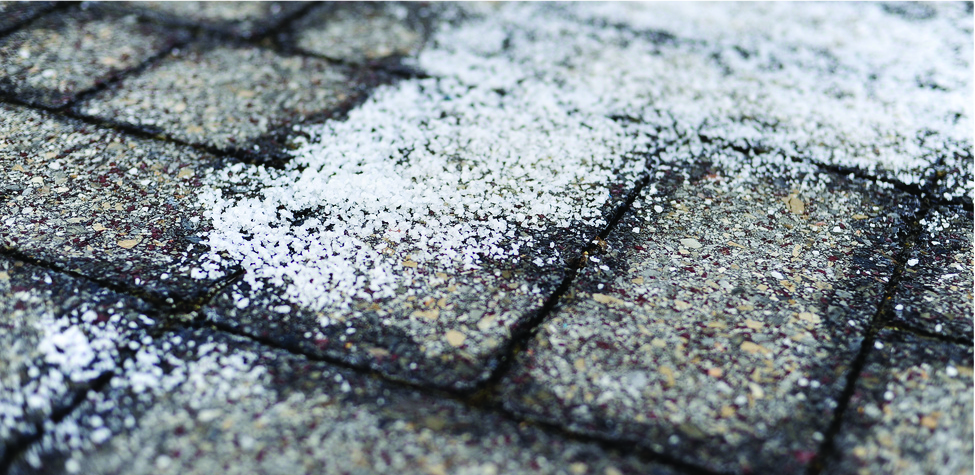Figure 4. Rock salt (NaCl), calcium chloride (CaCl2), or a mixture of the two are used to melt ice. (credit: modification of work by Eddie Welker)

The decrease in freezing point of a dilute solution compared to that of the pure solvent, ΔTf, is called the freezing point depression and is directly proportional to the molal concentration of the solute${\Delta}T_{\text{f}} = K_{\text{f}}m$

where m is the molal concentration of the solute in the solvent and Kf is called the freezing point depression constant (or cryoscopic constant). Just as for boiling point elevation constants, these are characteristic properties whose values depend on the chemical identity of the solvent. Values of Kf for several solvents are listed in Table 2.

### Colligative Properties and De-Icing

Sodium chloride and its group 2 analogs calcium and magnesium chloride are often used to de-ice roadways and sidewalks, due to the fact that a solution of any one of these salts will have a freezing point lower than 0 °C, the freezing point of pure water. The group 2 metal salts are frequently mixed with the cheaper and more readily available sodium chloride (“rock salt”) for use on roads, since they tend to be somewhat less corrosive than the NaCl, and they provide a larger depression of the freezing point, since they dissociate to yield three particles per formula unit, rather than two particles like the sodium chloride.

Because these ionic compounds tend to hasten the corrosion of metal, they would not be a wise choice to use in antifreeze for the radiator in your car or to de-ice a plane prior to takeoff. For these applications, covalent compounds, such as ethylene or propylene glycol, are often used. The glycols used in radiator fluid not only lower the freezing point of the liquid, but they elevate the boiling point, making the fluid useful in both winter and summer. Heated glycols are often sprayed onto the surface of airplanes prior to takeoff in inclement weather in the winter to remove ice that has already formed and prevent the formation of more ice, which would be particularly dangerous if formed on the control surfaces of the aircraft (Figure 5).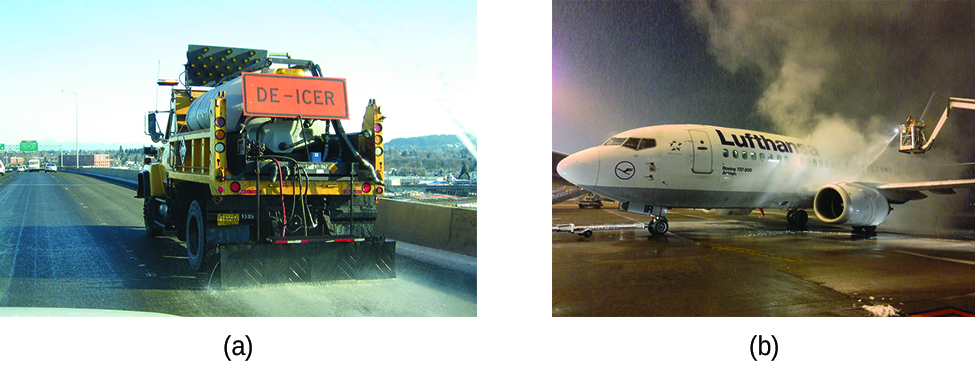Figure 5. Freezing point depression is exploited to remove ice from (a) roadways and (b) the control surfaces of aircraft.

# Phase Diagram for an Aqueous Solution of a Nonelectrolyte

The colligative effects on vapor pressure, boiling point, and freezing point described in the previous section are conveniently summarized by comparing the phase diagrams for a pure liquid and a solution derived from that liquid. Phase diagrams for water and an aqueous solution are shown in Figure 6.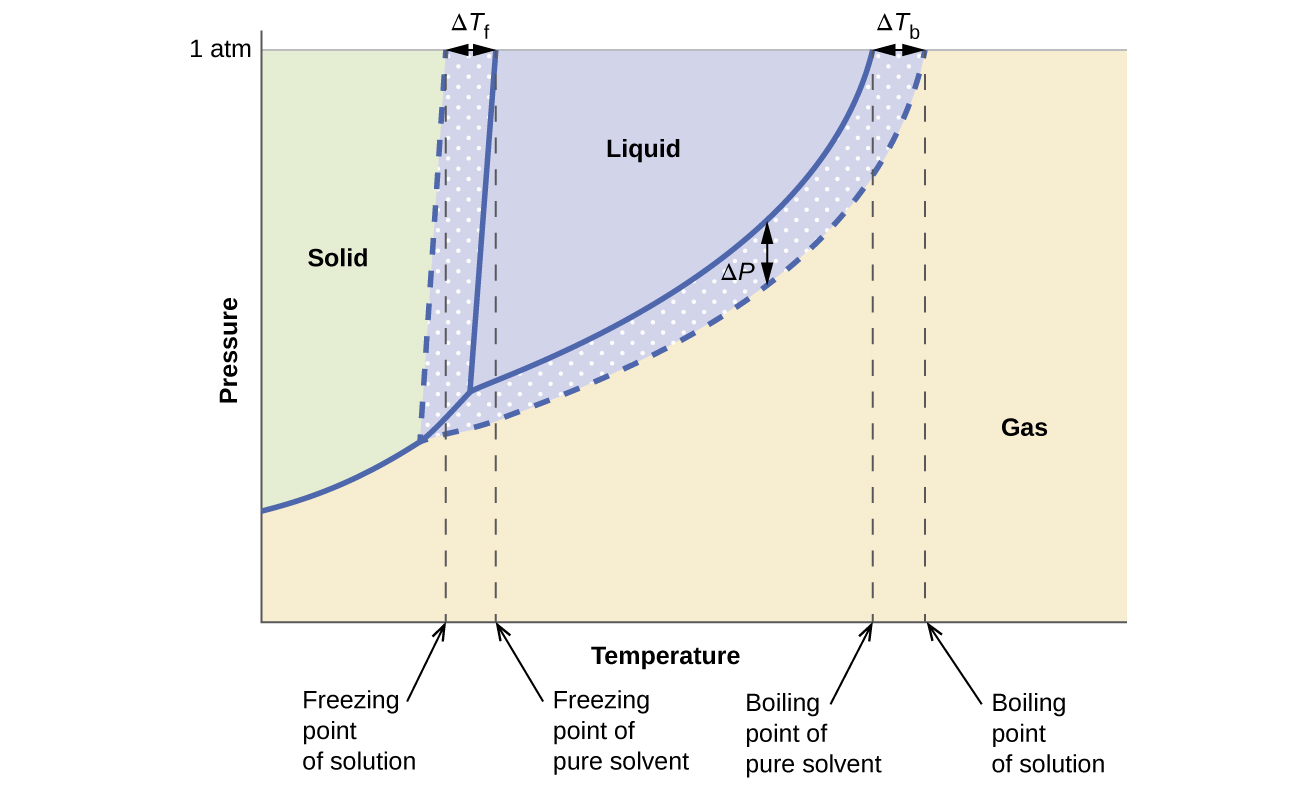Figure 6. These phase diagrams show water (solid curves) and an aqueous solution of nonelectrolyte (dashed curves).

The liquid-vapor curve for the solution is located beneath the corresponding curve for the solvent, depicting the vapor pressure lowering, ΔP, that results from the dissolution of nonvolatile solute. Consequently, at any given pressure, the solution’s boiling point is observed at a higher temperature than that for the pure solvent, reflecting the boiling point elevation, ΔTb, associated with the presence of nonvolatile solute. The solid-liquid curve for the solution is displaced left of that for the pure solvent, representing the freezing point depression, ΔTb, that accompanies solution formation. Finally, notice that the solid-gas curves for the solvent and its solution are identical. This is the case for many solutions comprising liquid solvents and nonvolatile solutes. Just as for vaporization, when a solution of this sort is frozen, it is actually just the solvent molecules that undergo the liquid-to-solid transition, forming pure solid solvent that excludes solute species. The solid and gaseous phases, therefore, are composed solvent only, and so transitions between these phases are not subject to colligative effects.

# Osmosis and Osmotic Pressure of Solutions

A number of natural and synthetic materials exhibit selective permeation, meaning that only molecules or ions of a certain size, shape, polarity, charge, and so forth, are capable of passing through (permeating) the material. Biological cell membranes provide elegant examples of selective permeation in nature, while dialysis tubing used to remove metabolic wastes from blood is a more simplistic technological example. Regardless of how they may be fabricated, these materials are generally referred to as semipermeable membranes.

Consider the apparatus illustrated in Figure 7, in which samples of pure solvent and a solution are separated by a membrane that only solvent molecules may permeate. Solvent molecules will diffuse across the membrane in both directions. Since the concentration of solvent is greater in the pure solvent than the solution, these molecules will diffuse from the solvent side of the membrane to the solution side at a faster rate than they will in the reverse direction. The result is a net transfer of solvent molecules from the pure solvent to the solution. Diffusion-driven transfer of solvent molecules through a semipermeable membrane is a process known as osmosis.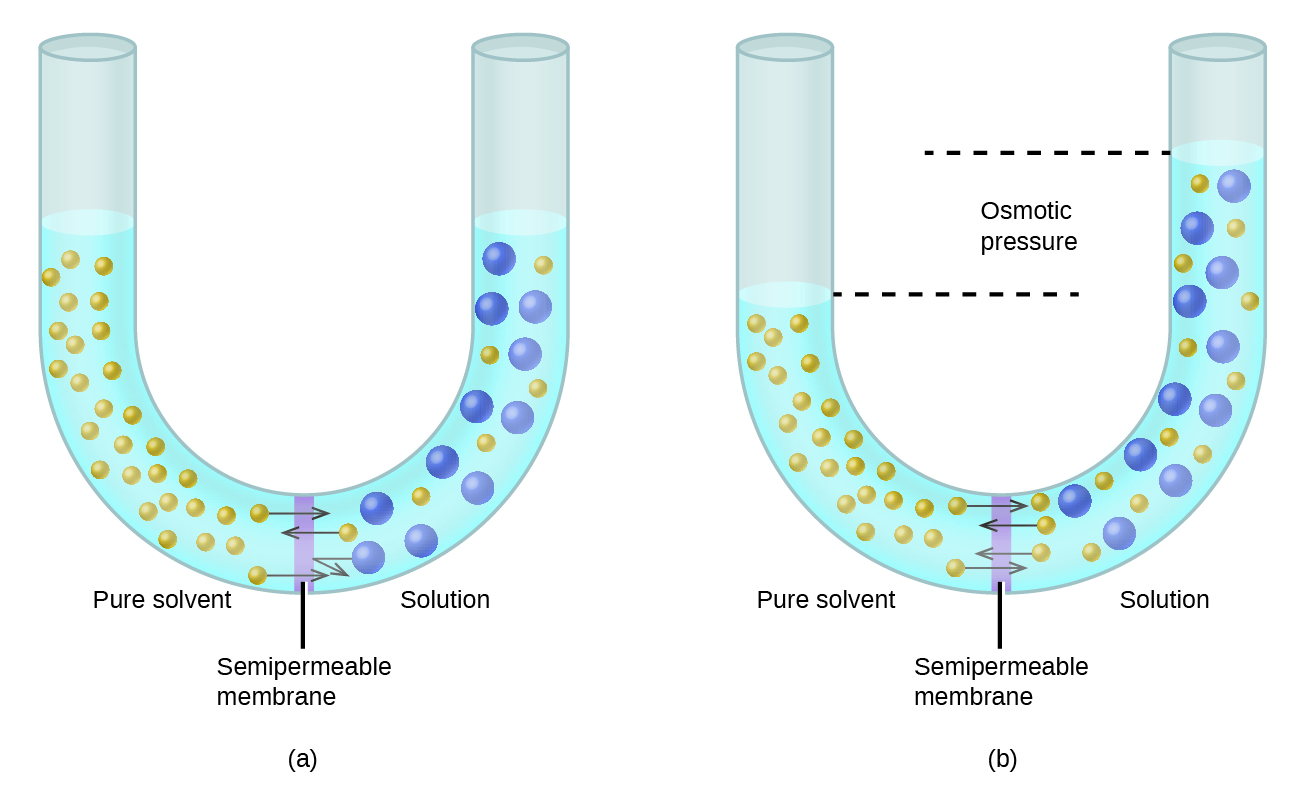Figure 7. Osmosis results in the transfer of solvent molecules from a sample of low (or zero) solute concentration to a sample of higher solute concentration.

When osmosis is carried out in an apparatus like that shown in Figure 7, the volume of the solution increases as it becomes diluted by accumulation of solvent. This causes the level of the solution to rise, increasing its hydrostatic pressure (due to the weight of the column of solution in the tube) and resulting in a faster transfer of solvent molecules back to the pure solvent side. When the pressure reaches a value that yields a reverse solvent transfer rate equal to the osmosis rate, bulk transfer of solvent ceases. This pressure is called the osmotic pressure (Π) of the solution. The osmotic pressure of a dilute solution is related to its solute molarity, M, and absolute temperature, T, according to the equation$\it{{\Pi}} = MRT$

where R is the universal gas constant.

If a solution is placed in an apparatus like the one shown in Figure 8, applying pressure greater than the osmotic pressure of the solution reverses the osmosis and pushes solvent molecules from the solution into the pure solvent. This technique of reverse osmosis is used for large-scale desalination of seawater and on smaller scales to produce high-purity tap water for drinking.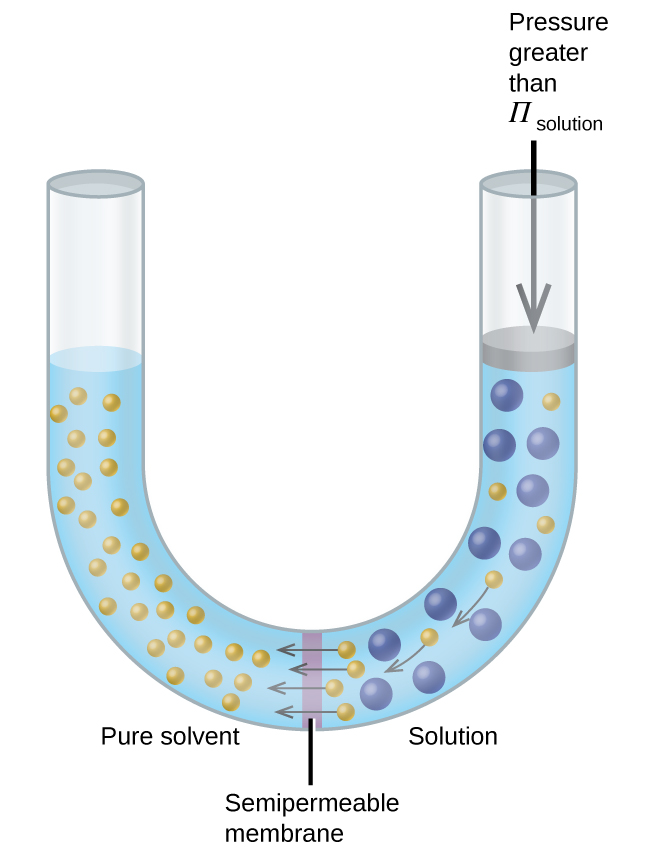Figure 8. Applying a pressure greater than the osmotic pressure of a solution will reverse osmosis. Solvent molecules from the solution are pushed into the pure solvent.

### Reverse Osmosis Water Purification

In the process of osmosis, diffusion serves to move water through a semipermeable membrane from a less concentrated solution to a more concentrated solution. Osmotic pressure is the amount of pressure that must be applied to the more concentrated solution to cause osmosis to stop. If greater pressure is applied, the water will go from the more concentrated solution to a less concentrated (more pure) solution. This is called reverse osmosis. Reverse osmosis (RO) is used to purify water in many applications, from desalination plants in coastal cities, to water-purifying machines in grocery stores (Figure 9), and smaller reverse-osmosis household units. With a hand-operated pump, small RO units can be used in third-world countries, disaster areas, and in lifeboats. Our military forces have a variety of generator-operated RO units that can be transported in vehicles to remote locations.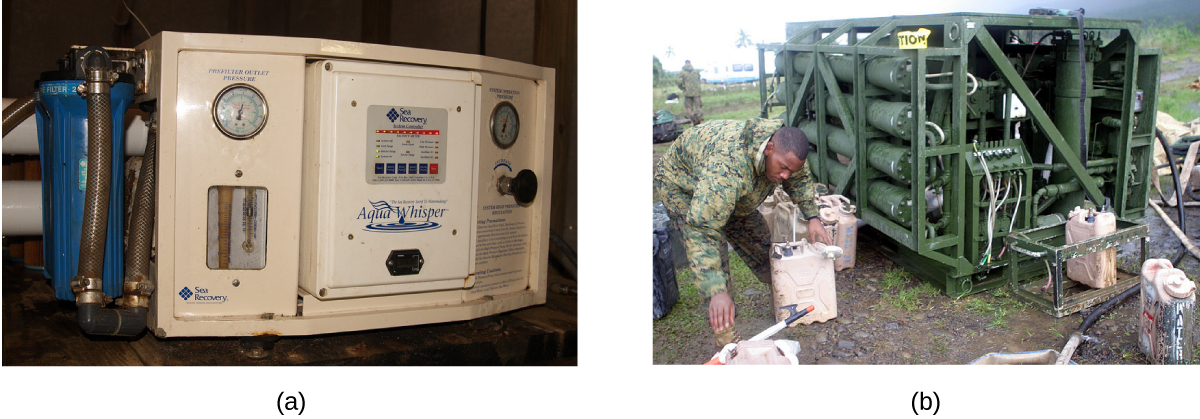Figure 9. Reverse osmosis systems for purifying drinking water are shown here on (a) small and (b) large scales. (credit a: modification of work by Jerry Kirkhart; credit b: modification of work by Willard J. Lathrop) Colligative Properties of Electrolytes As noted previously in this module, the colligative properties of a solution depend only on the number, not on the kind, of solute species dissolved. For example, 1 mole of any nonelectrolyte dissolved in 1 kilogram of solvent produces the same lowering of the freezing point as does 1 mole of any other nonelectrolyte. However, 1 mole of sodium chloride (an electrolyte) forms 2 moles of ions when dissolved in solution. Each individual ion produces the same effect on the freezing point as a single molecule does.

Examples of osmosis are evident in many biological systems because cells are surrounded by semipermeable membranes. Carrots and celery that have become limp because they have lost water can be made crisp again by placing them in water. Water moves into the carrot or celery cells by osmosis. A cucumber placed in a concentrated salt solution loses water by osmosis and absorbs some salt to become a pickle. Osmosis can also affect animal cells. Solute concentrations are particularly important when solutions are injected into the body. Solutes in body cell fluids and blood serum give these solutions an osmotic pressure of approximately 7.7 atm. Solutions injected into the body must have the same osmotic pressure as blood serum; that is, they should be isotonic with blood serum. If a less concentrated solution, a hypotonicsolution, is injected in sufficient quantity to dilute the blood serum, water from the diluted serum passes into the blood cells by osmosis, causing the cells to expand and rupture. This process is called hemolysis. When a more concentrated solution, a hypertonic solution, is injected, the cells lose water to the more concentrated solution, shrivel, and possibly die in a process called crenation. These effects are illustrated in Figure 10.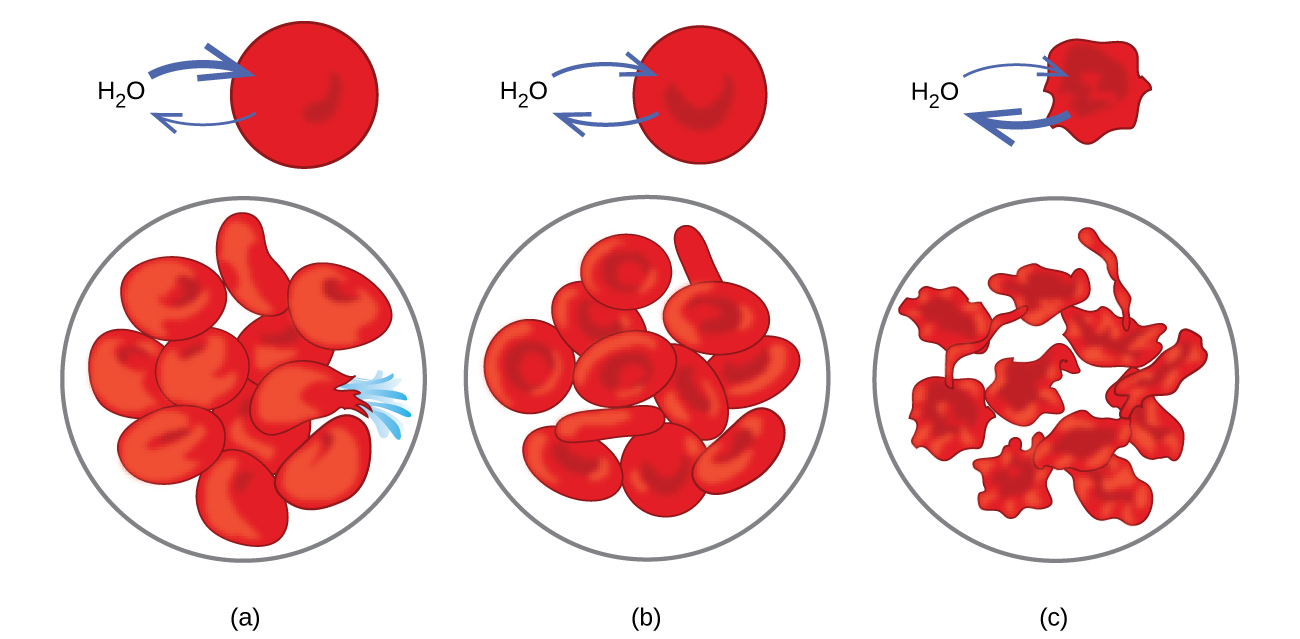Figure 10. Red blood cell membranes are water permeable and will (a) swell and possibly rupture in a hypotonic solution; (b) maintain normal volume and shape in an isotonic solution; and (c) shrivel and possibly die in a hypertonic solution. (credit a/b/c: modifications of work by “LadyofHats”/Wikimedia commons)

# Determination of Molar Masses

Osmotic pressure and changes in freezing point, boiling point, and vapor pressure are directly proportional to the concentration of solute present. Consequently, we can use a measurement of one of these properties to determine the molar mass of the solute from the measurements.

Assuming complete dissociation, a 1.0 m aqueous solution of NaCl contains 1.0 mole of ions (1.0 mol Na+ and 1.0 mol Cl) per each kilogram of water, and its freezing point depression is expected to be${\Delta}T_{\text{f}} = 2.0\;\text{mol\;ions/kg\;water}\;\times\;1.86\;^{\circ}\text{C\;kg\;water/mol\;ion} = 3.7\;^{\circ}\text{C}$.

When this solution is actually prepared and its freezing point depression measured, however, a value of 3.4 °C is obtained. Similar discrepancies are observed for other ionic compounds, and the differences between the measured and expected colligative property values typically become more significant as solute concentrations increase. These observations suggest that the ions of sodium chloride (and other strong electrolytes) are not completely dissociated in solution.

To account for this and avoid the errors accompanying the assumption of total dissociation, an experimentally measured parameter named in honor of Nobel Prize-winning German chemist Jacobus Henricus van’t Hoff is used. The van’t Hoff factor (i) is defined as the ratio of solute particles in solution to the number of formula units dissolved:$i = \frac{\text{moles\;of\;particles\;in\;solution}}{\text{moles\;of\;formula\;units\;dissolved}}$

Values for measured van’t Hoff factors for several solutes, along with predicted values assuming complete dissociation, are shown in Table 3.

Electrolyte Particles in Solution i (Predicted) i (Measured)
HCl H+, Cl 2 1.9
NaCl Na+, Cl 2 1.9
MgSO4 Mg2+, SO42- 2 1.3
MgCl2 Mg2+, 2Cl 3 2.7
FeCl3 Fe3+, 3Cl 4 3.4
glucose C12H22O11 1 1.0
Table 3. Expected and Observed van’t Hoff Factors for Several 0.050 m Aqueous Electrolyte Solutions

In 1923, the chemists Peter Debye and Erich Hückel proposed a theory to explain the apparent incomplete ionization of strong electrolytes. They suggested that although interionic attraction in an aqueous solution is very greatly reduced by solvation of the ions and the insulating action of the polar solvent, it is not completely nullified. The residual attractions prevent the ions from behaving as totally independent particles (Figure 11). In some cases, a positive and negative ion may actually touch, giving a solvated unit called an ion pair. Thus, the activity, or the effective concentration, of any particular kind of ion is less than that indicated by the actual concentration. Ions become more and more widely separated the more dilute the solution, and the residual interionic attractions become less and less. Thus, in extremely dilute solutions, the effective concentrations of the ions (their activities) are essentially equal to the actual concentrations. Note that the van’t Hoff factors for the electrolytes in Table 3 are for 0.05 msolutions, at which concentration the value of i for NaCl is 1.9, as opposed to an ideal value of 2.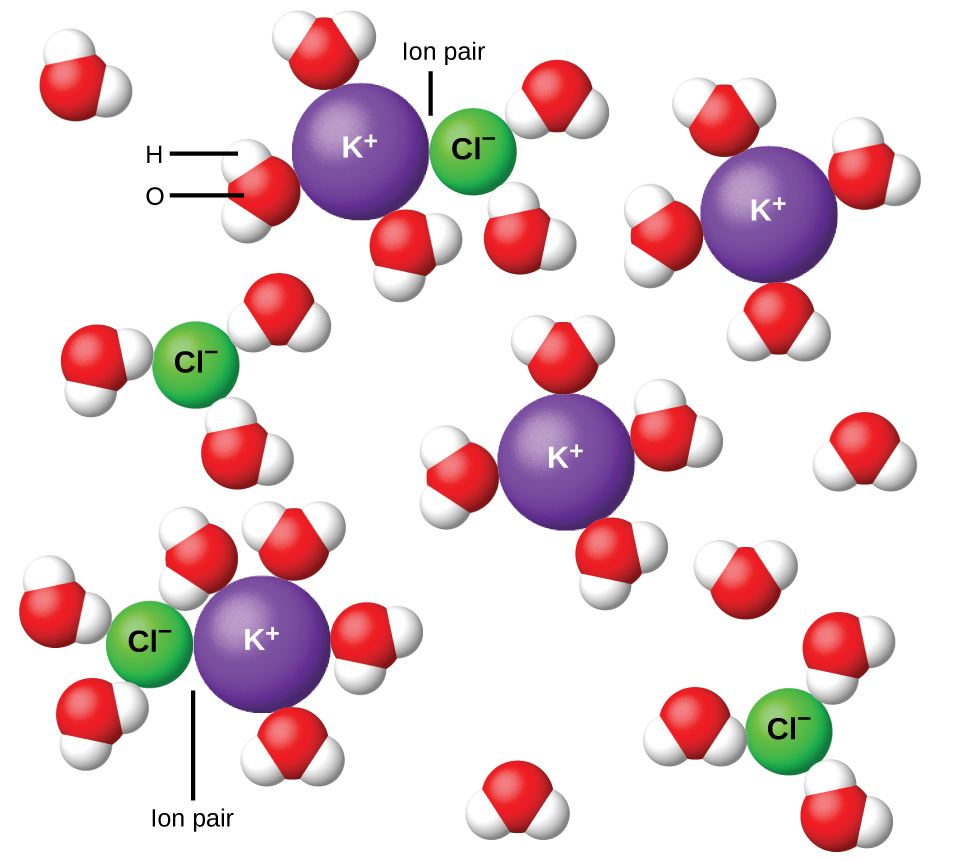Figure 11. Ions become more and more widely separated the more dilute the solution, and the residual interionic attractions become less.

# Key Concepts and Summary

Properties of a solution that depend only on the concentration of solute particles are called colligative properties. They include changes in the vapor pressure, boiling point, and freezing point of the solvent in the solution. The magnitudes of these properties depend only on the total concentration of solute particles in solution, not on the type of particles. The total concentration of solute particles in a solution also determines its osmotic pressure. This is the pressure that must be applied to the solution to prevent diffusion of molecules of pure solvent through a semipermeable membrane into the solution. Ionic compounds may not completely dissociate in solution due to activity effects, in which case observed colligative effects may be less than predicted.

# Key Equations

•$(P_{\text{A}} = X_{\text{A}}P_{\text{A}}^{\circ})$
•$P_{\text{solution}} = \sum\limits_{i}\;P_i = \sum\limits_{i}\;X_iP_i^{\circ}$
•$P_{\text{solution}} = X_{\text{solvent}}P_{\text{solvent}}^{\circ}$
• ΔTb = Kbm
• ΔTf = Kfm
• Π = MRT

Assuming complete dissociation, a 1.0 m aqueous solution of NaCl contains 1.0 mole of ions (1.0 mol Na+ and 1.0 mol Cl) per each kilogram of water, and its freezing point depression is expected to be${\Delta}T_{\text{f}} = 2.0\;\text{mol\;ions/kg\;water}\;\times\;1.86\;^{\circ}\text{C\;kg\;water/mol\;ion} = 3.7\;^{\circ}\text{C}$.

When this solution is actually prepared and its freezing point depression measured, however, a value of 3.4 °C is obtained. Similar discrepancies are observed for other ionic compounds, and the differences between the measured and expected colligative property values typically become more significant as solute concentrations increase. These observations suggest that the ions of sodium chloride (and other strong electrolytes) are not completely dissociated in solution.

To account for this and avoid the errors accompanying the assumption of total dissociation, an experimentally measured parameter named in honor of Nobel Prize-winning German chemist Jacobus Henricus van’t Hoff is used. The van’t Hoff factor (i) is defined as the ratio of solute particles in solution to the number of formula units dissolved:$i = \frac{\text{moles\;of\;particles\;in\;solution}}{\text{moles\;of\;formula\;units\;dissolved}}$

Values for measured van’t Hoff factors for several solutes, along with predicted values assuming complete dissociation, are shown in Table 3.

Electrolyte Particles in Solution i (Predicted) i (Measured)
HCl H+, Cl 2 1.9
NaCl Na+, Cl 2 1.9
MgSO4 Mg2+, SO42- 2 1.3
MgCl2 Mg2+, 2Cl 3 2.7
FeCl3 Fe3+, 3Cl 4 3.4
glucose C12H22O11 1 1.0
Table 3. Expected and Observed van’t Hoff Factors for Several 0.050 m Aqueous Electrolyte Solutions

In 1923, the chemists Peter Debye and Erich Hückel proposed a theory to explain the apparent incomplete ionization of strong electrolytes. They suggested that although interionic attraction in an aqueous solution is very greatly reduced by solvation of the ions and the insulating action of the polar solvent, it is not completely nullified. The residual attractions prevent the ions from behaving as totally independent particles (Figure 11). In some cases, a positive and negative ion may actually touch, giving a solvated unit called an ion pair. Thus, the activity, or the effective concentration, of any particular kind of ion is less than that indicated by the actual concentration. Ions become more and more widely separated the more dilute the solution, and the residual interionic attractions become less and less. Thus, in extremely dilute solutions, the effective concentrations of the ions (their activities) are essentially equal to the actual concentrations. Note that the van’t Hoff factors for the electrolytes in Table 3 are for 0.05 msolutions, at which concentration the value of i for NaCl is 1.9, as opposed to an ideal value of 2.Figure 11. Ions become more and more widely separated the more dilute the solution, and the residual interionic attractions become less.

# Key Concepts and Summary

Properties of a solution that depend only on the concentration of solute particles are called colligative properties. They include changes in the vapor pressure, boiling point, and freezing point of the solvent in the solution. The magnitudes of these properties depend only on the total concentration of solute particles in solution, not on the type of particles. The total concentration of solute particles in a solution also determines its osmotic pressure. This is the pressure that must be applied to the solution to prevent diffusion of molecules of pure solvent through a semipermeable membrane into the solution. Ionic compounds may not completely dissociate in solution due to activity effects, in which case observed colligative effects may be less than predicted.

# Key Equations

•$(P_{\text{A}} = X_{\text{A}}P_{\text{A}}^{\circ})$
•$P_{\text{solution}} = \sum\limits_{i}\;P_i = \sum\limits_{i}\;X_iP_i^{\circ}$
•$P_{\text{solution}} = X_{\text{solvent}}P_{\text{solvent}}^{\circ}$
• ΔTb = Kbm
• ΔTf = Kfm
• Π = MRT

## STEM Elearning

We at FAWE have built this platform to aid learners, trainers and mentors get practical help with content, an interactive platform and tools to power their teaching and learning of STEM subjects, more

#### How to find your voice as a woman in Africa

© FAWE, Powered by: Yaaka DN.